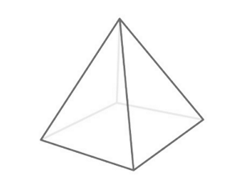Surface Area of A Square Pyramid Calculator

Side of the base
Slant height
Area Of Square Pyramid Square UnitsThe Surface Area of a Square Pyramid Calculator an online tool which shows Surface Area of a Square Pyramid for the given input. Byju's Surface Area of a Square Pyramid Calculator is a tool
which makes calculations very simple and interesting. If an input is given then it can easily show the result for the given number.